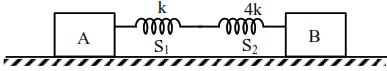# In the reported figure,`
Question:

In the reported figure, two bodies $A$ and $B$ of masses $200 \mathrm{~g}$ and $800 \mathrm{~g}$ are attached with the system of springs. Springs are kept in a stretched position with some extension when the system is released. The horizontal surface is assumed to be frictionless. The angular frequency will be _____________ $\mathrm{rad} / \mathrm{s}$ when $\mathrm{k}=20 \mathrm{~N} / \mathrm{m}$.Solution:

$\omega=\sqrt{\frac{k_{e q}}{\mu}}$

$\mu=$ reduced mass

springs are in series connection

$\mathrm{k}_{\mathrm{cq}}=\frac{\mathrm{k}_{1} \mathrm{k}_{2}}{\mathrm{k}_{1}+\mathrm{k}_{2}}$

$\mathrm{k}_{\mathrm{cq}}=\frac{\mathrm{k} \times 4 \mathrm{k}}{5 \mathrm{k}}=\frac{4 \mathrm{k}}{5}$

$\mathrm{k}_{\mathrm{cq}}=\frac{4 \times 20}{5} \mathrm{~N} / \mathrm{m}=16 \mathrm{~N} / \mathrm{m}$

$\mu=\frac{\mathrm{m}_{1} \mathrm{~m}_{2}}{\mathrm{~m}_{1}+\mathrm{m}_{2}}=\frac{0.2 \times 0.8}{0.2+0.8}=0.16 \mathrm{~kg}$

$\omega=\sqrt{\frac{16}{0.16}}=\sqrt{100}=10$